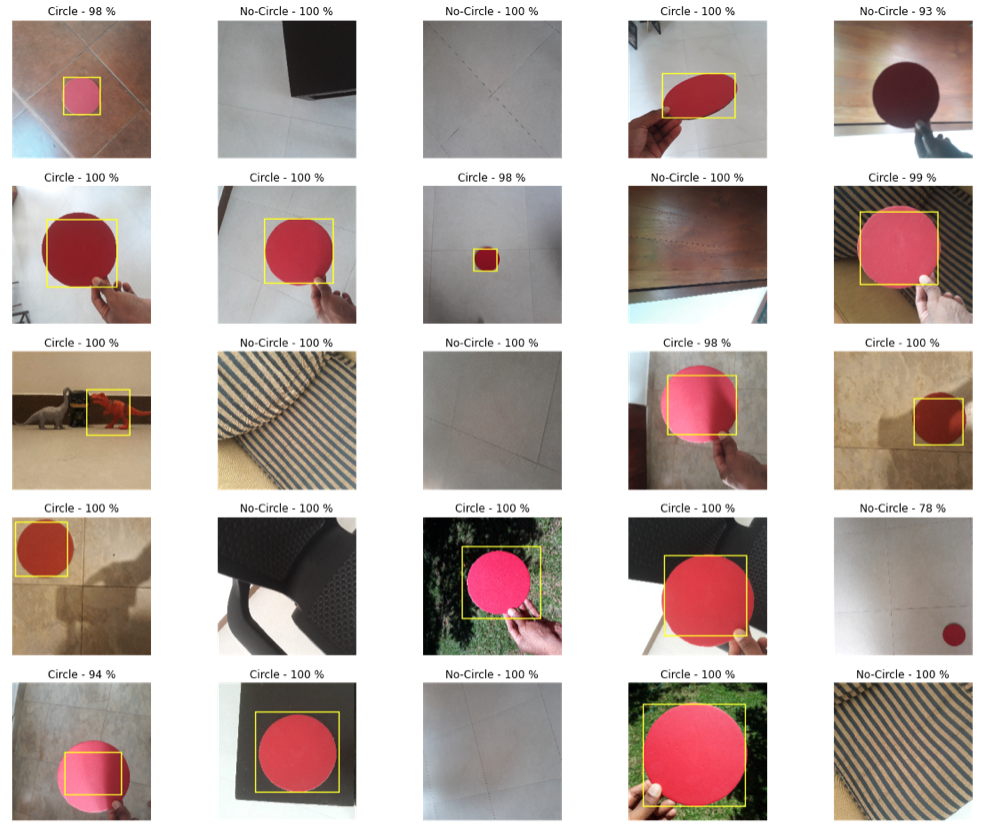# 利用包围盒回归在TensorFlow中构建目标检测器

## 要检测的对象## 模型的体系结构

• 无论图像中是否包含对象
• 物体的确切位置在哪里？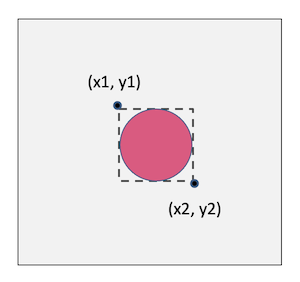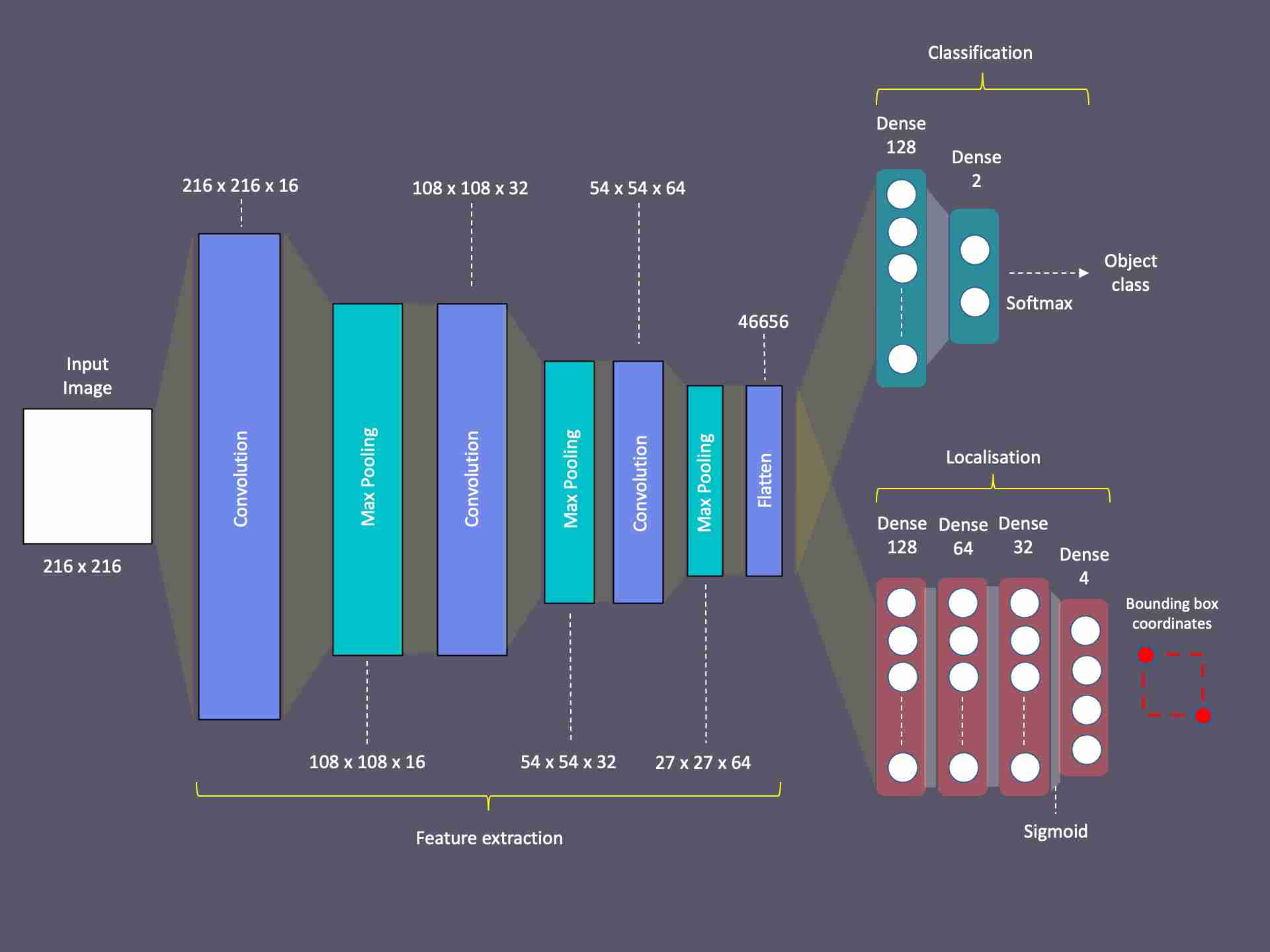## 数据准备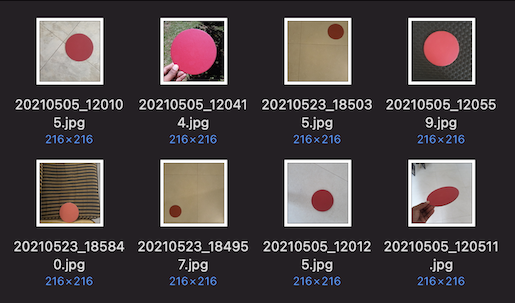## 图像标注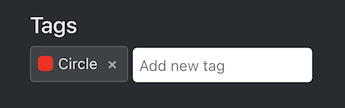## 组织文件夹结构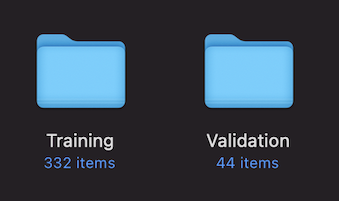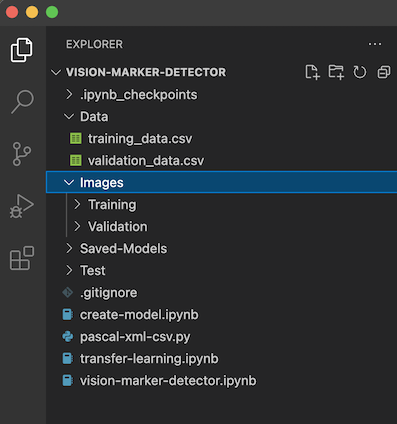## 创建数据集

train_images = np.array(train_images)train_targets = np.array(train_targets)train_labels = np.array(train_labels)

## 构建模型

import matplotlib.pyplot as pltimport numpy as npimport osimport PILimport tensorflow as tf

width = 216height = 216num_classes = 2

model = tf.keras.Model(input_layer,           outputs=[classifier_branch,locator_branch])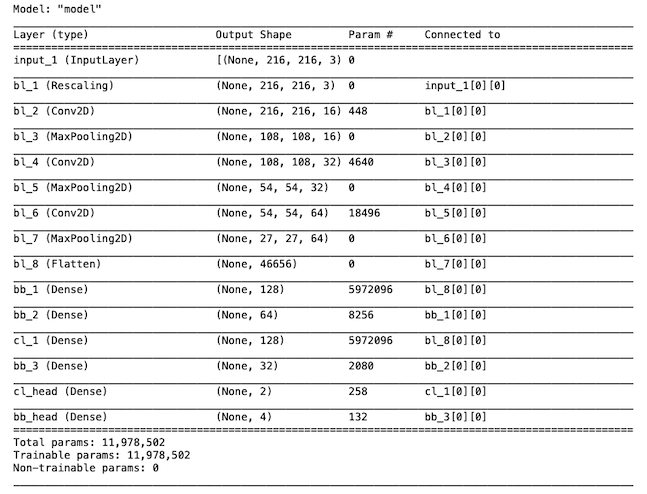## 编译模型

model.compile(loss=losses, optimizer='Adam', metrics=['accuracy'])

## 本地化-包围盒回归模型的训练trainTargets = {    "cl_head": train_labels,    "bb_head": train_targets}

history = model.fit(train_images, trainTargets,             validation_data=(validation_images, validationTargets),             batch_size=4,             epochs=training_epochs,             shuffle=True,             verbose=1)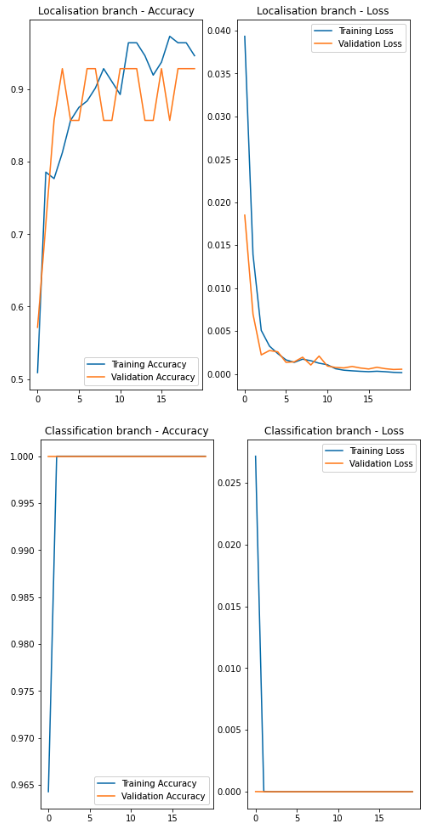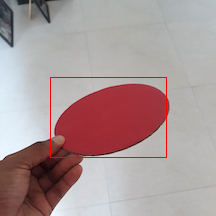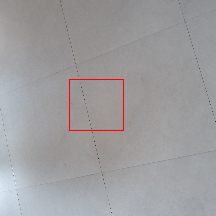## 训练模型进行分类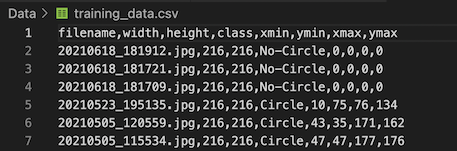for layer in model.layers:    if layer.name.startswith('bl_'):        layer.trainable = False        for layer in model.layers:    if layer.name.startswith('bb_'):        layer.trainable = False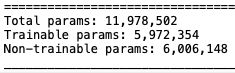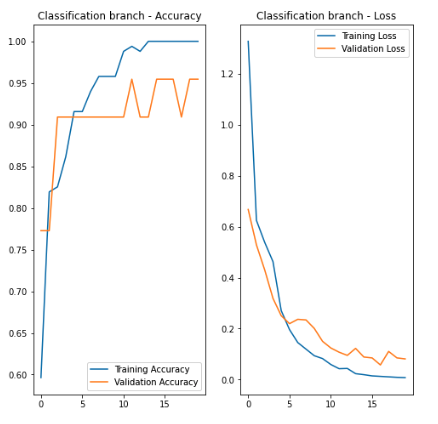## 使用该模型进行目标检测